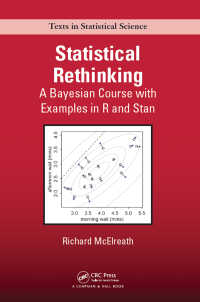## 電子書籍詳細Statistical Rethinking : A Bayesian Course with Examples in R and Stan

1

(Chapman & Hall/CRC Texts in Statistical Science)

McElreath, Richard

Chapman and Hall/CRC 2018/01

ISBN: 9781482253443
eISBN: 9781482253481
KNPID: EY00224213

この商品はBookWeb Proでのお取り扱いができません。恐れ入りますがご了承下さい。

ファイルフォーマット:
ファイルサイズ:
デバイス:ご購入を希望される方は、下のリンクをクリックしてください。

### Full Description

Statistical Rethinking: A Bayesian Course with Examples in R and Stan builds readers’ knowledge of and confidence in statistical modeling. Reflecting the need for even minor programming in today’s model-based statistics, the book pushes readers to perform step-by-step calculations that are usually automated. This unique computational approach ensures that readers understand enough of the details to make reasonable choices and interpretations in their own modeling work.

The text presents generalized linear multilevel models from a Bayesian perspective, relying on a simple logical interpretation of Bayesian probability and maximum entropy. It covers from the basics of regression to multilevel models. The author also discusses measurement error, missing data, and Gaussian process models for spatial and network autocorrelation.

By using complete R code examples throughout, this book provides a practical foundation for performing statistical inference. Designed for both PhD students and seasoned professionals in the natural and social sciences, it prepares them for more advanced or specialized statistical modeling.

Web Resource
The book is accompanied by an R package (rethinking) that is available on the author’s website and GitHub. The two core functions (map and map2stan) of this package allow a variety of statistical models to be constructed from standard model formulas.

The Golem of Prague
Statistical golems
Statistical rethinking
Three tools for golem engineering
Summary

Small Worlds and Large Worlds
The garden of forking data
Building a model
Components of the model
Making the model go
Summary
Practice

Sampling the Imaginary
Sampling from a grid-approximate posterior
Sampling to summarize
Sampling to simulate prediction
Summary
Practice

Linear Models
Why normal distributions are normal
A language for describing models
A Gaussian model of height
Polynomial regression
Summary
Practice

Multivariate Linear Models
Spurious association
Categorical variables
Ordinary least squares and lm
Summary
Practice

Overfitting, Regularization, and Information Criteria
The problem with parameters
Information theory and model performance
Regularization
Information criteria
Using information criteria
Summary
Practice

Interactions
Building an interaction
Symmetry of the linear interaction
Continuous interactions
Interactions in design formulas
Summary
Practice

Markov Chain Monte Carlo
Good King Markov and His island kingdom
Markov chain Monte Carlo
Easy HMC: map2stan
Care and feeding of your Markov chain
Summary
Practice

Big Entropy and the Generalized Linear Model
Maximum entropy
Generalized linear models
Maximum entropy priors
Summary

Counting and Classification
Binomial regression
Poisson regression
Other count regressions
Summary
Practice

Monsters and Mixtures
Ordered categorical outcomes
Zero-inflated outcomes
Over-dispersed outcomes
Summary
Practice

Multilevel Models
Varying effects and the underfitting/overfitting trade-off
More than one type of cluster
Multilevel posterior predictions
Summary
Practice

Varying slopes by construction